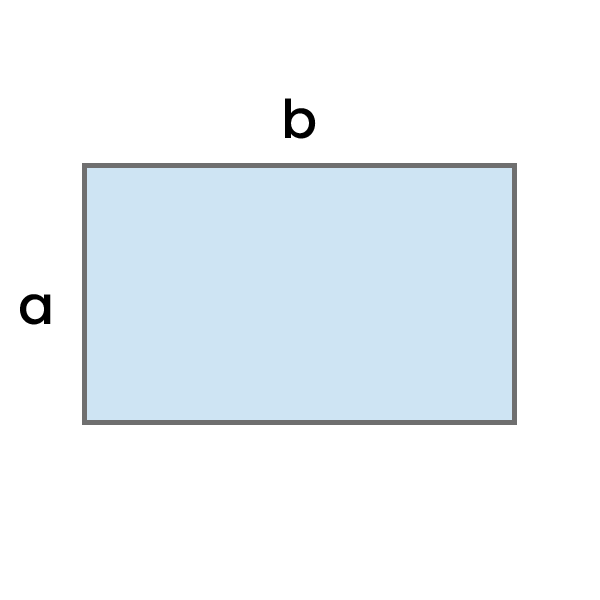# Perimeter of a Rectangle Calculator

Calculate the perimeter of a rectangle based on the entered height and width in the chosen units.Result:

## How to use this calculator?

1. Enter the length of the rectangle in your chosen unit.
2. Enter the width of the rectangle in your chosen unit.
3. Check the result you've obtained. If necessary, adjust its unit to your preference.

You can also see the detailed calculation process below the "result" field.

## How to calculate the Perimeter of a Rectangle?

To calculate the area of a rectangle, you need to add together both lengths of side "a" and both lengths of side "b".

Perimeter of a Rectangle = 2 * a + 2 * b
Example: Calculate the perimeter of a rectangle with a height of 7 cm and width of 12 cm.

P = 2 * a + 2 * b

P = 2 * 7 cm + 2 * 12 cm

P = 14 cm + 24 cm

P = 38 cm